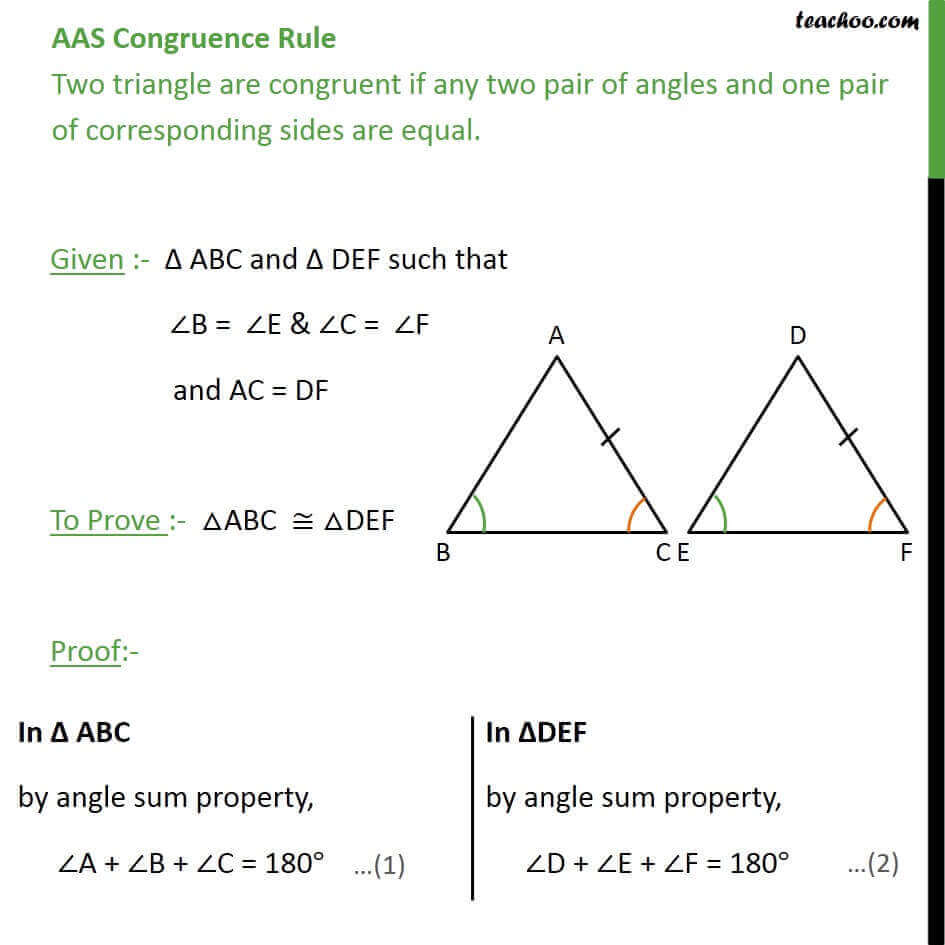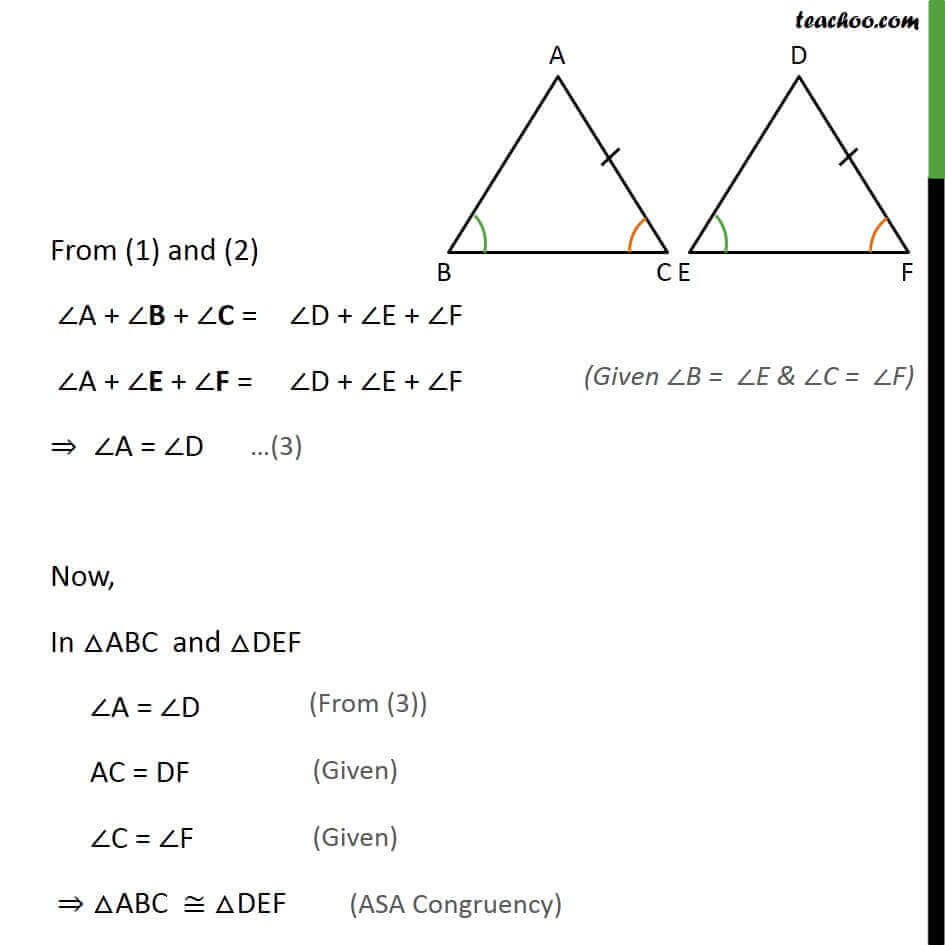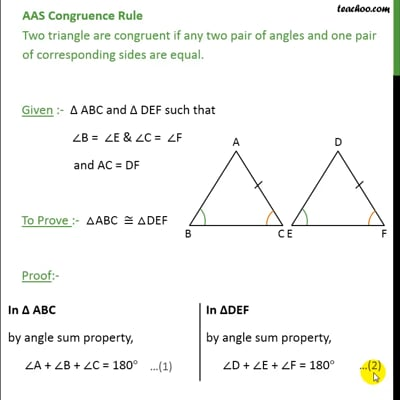Theorems

Chapter 7 Class 9 Triangles
Serial order wiseThis video is only available for Teachoo black users

Solve all your doubts with Teachoo Black (new monthly pack available now!)

### Transcript

AAS Congruence Rule Two triangle are congruent if any two pair of angles and one pair of corresponding sides are equal. Given :- Δ ABC and Δ DEF such that ∠B = ∠E & ∠C = ∠F and AC = DF To Prove :- △ABC ≅ △DEF Proof:- From (1) and (2) ∠A + ∠B + ∠C = ∠D + ∠E + ∠F ∠A + ∠E + ∠F = ∠D + ∠E + ∠F ⇒ ∠A = ∠D Now, In △ABC and △DEF ∠A = ∠D AC = DF ∠C = ∠F ⇒ △ABC ≅ △DEF